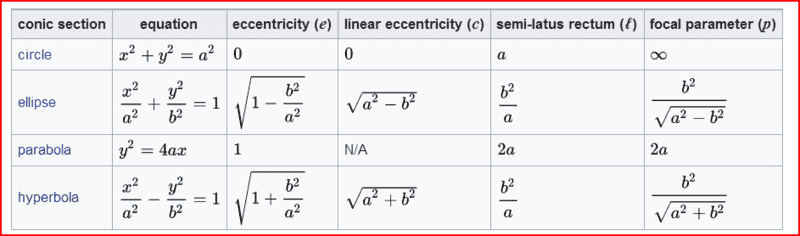# Y=cx[L−x], The value of the constant c for a perfect circle

• member 634857

## Homework Statement

For the equation y=cx[L−x] say for a circle with the value of L at 100 meters and the value of x at 25 meters.
What would be the value of the constant c for a perfect circle.

3. Attempt at the Solution:
I can approximate and graph this with different values of c however I'm really interested in what is the precise value of c for a perfect circle. I would have to at this point just plug in "1" and see what comes out which really isn't where I wanted to be after reading:
http://www.engineeringwiki.org/wiki/Arch_Structures

Thank You!

Last edited by a moderator:

## Homework Statement

For the equation y=cx[L−x] say for a circle with the value of L at 100 meters and the value of x at 25 meters.
What would be the value of the constant c for a perfect circle.

3. Attempt at the Solution:
I can approximate and graph this with different values of c however I'm really interested in what is the precise value of c for a perfect circle. I would have to at this point just plug in "1" and see what comes out which really isn't where I wanted to be after reading:
http://www.engineeringwiki.org/wiki/Arch_Structures

Thank You!
Are you sure that's the equation? Looks more parabolic to me than a circle...

I agree. That isn’t a circle. Also, what could it possibly mean about being a circle at a particular value of x? This problem seems to be nonsense

A circle is simply a form then of conic and so is a parabola. They should overlap at some point. ?

That's OK I can probably figure this out myself without help!

A circle is simply a form then of conic and so is a parabola. They should overlap at some point. ?
The equations have different forms. Do you see how the powers of the variables are different for the different conic sections?

Do you see how the powers of the variables are different for the different conic sections?
From the Wikipedia article on conic sections:

https://en.wikipedia.org/wiki/Conic_section#### Attachments

•SammyS
I apologize but that doesn't answer my question as well I was insulted first and your the insults I'm afraid. I wish to dissolve my account at this point. The services of your members are no longer required by me.

Thread is closed for a bit...

A circle is simply a form then of conic and so is a parabola. They should overlap at some point. ?
As already mentioned, the equations are different. The equation for a parabola is first degree in one variable and second degree in the other variable. For a circle, both variable occur to the second power. I don't know what you mean by "they should overlap at some point."

The equation that @berkeman shows in the table, ##y^2 = 4ax## is of a parabola that opens to the right (if a > 0). The equation ##x^2 = 4ay## is that of a parabola that opens upward.

Your equation, ##y = cx(L - x)## is a parabola that opens downward, assuming that both c and L are positive constants. There is no value of c that makes this the equation of a circle.

•berkeman
I was insulted first and your the insults I'm afraid
No, you weren't insulted. Members here were questioning the validity of the question you asked -- these were not insults, nor were they directed to you.